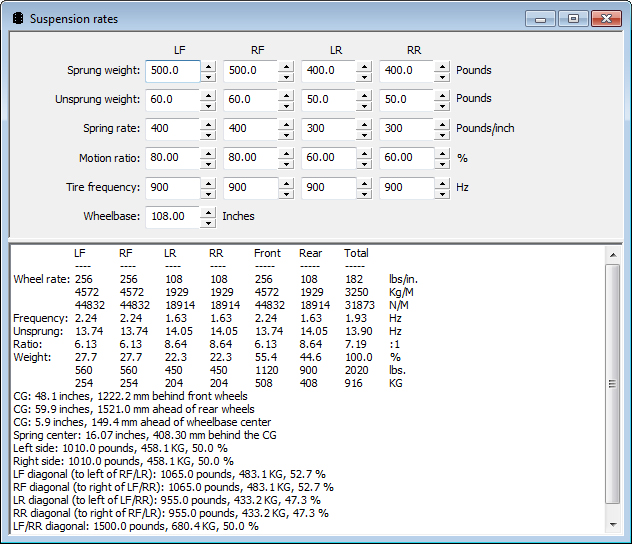## Suspension Weights Calculation

The Speed-Wiz Suspension Weights Calculation provides a wide variety of data based on the sprung and unsprung weights, spring rates, and motion ratios from each corner of a vehicle.Enter sprung weight and unsprung weight for each wheel, spring rate for each wheel, motion ratio for each wheel, and tire frequency for each wheel. Calculate wheel rates, wheel frequencies, unsprung weight, unsprung weight ratio, and total weight for each wheel, center of gravity position measured from front and wheel wheels, spring center in relation to center of gravity, left side weight, right side weight, LF diagonal weight (to left of RF/LR), RF diagonal weight (to right of LF/RR), LR diagonal weight (to left of LF/RR), RR diagonal weight (to right of RF/LR), LF/RR diagonal weight, and RF/LR diagonal weight.